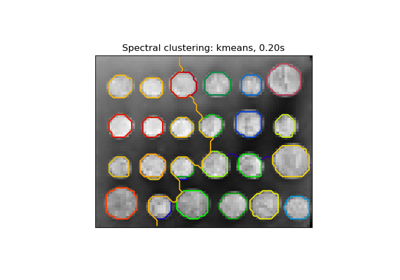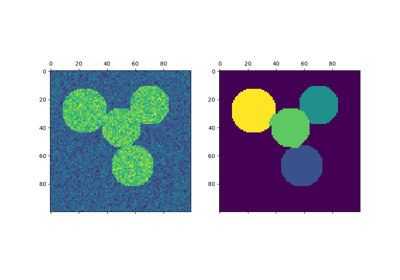# sklearn.cluster.spectral_clustering¶

sklearn.cluster.spectral_clustering(affinity, *, n_clusters=8, n_components=None, eigen_solver=None, random_state=None, n_init=10, eigen_tol='auto', assign_labels='kmeans', verbose=False)[source]

Apply clustering to a projection of the normalized Laplacian.

In practice Spectral Clustering is very useful when the structure of the individual clusters is highly non-convex or more generally when a measure of the center and spread of the cluster is not a suitable description of the complete cluster. For instance, when clusters are nested circles on the 2D plane.

If affinity is the adjacency matrix of a graph, this method can be used to find normalized graph cuts , .

Read more in the User Guide.

Parameters:
affinity{array-like, sparse matrix} of shape (n_samples, n_samples)

The affinity matrix describing the relationship of the samples to embed. Must be symmetric.

Possible examples:
• adjacency matrix of a graph,

• heat kernel of the pairwise distance matrix of the samples,

• symmetric k-nearest neighbours connectivity matrix of the samples.

n_clustersint, default=None

Number of clusters to extract.

n_componentsint, default=n_clusters

Number of eigenvectors to use for the spectral embedding.

eigen_solver{None, ‘arpack’, ‘lobpcg’, or ‘amg’}

The eigenvalue decomposition method. If None then 'arpack' is used. See  for more details regarding 'lobpcg'. Eigensolver 'amg' runs 'lobpcg' with optional Algebraic MultiGrid preconditioning and requires pyamg to be installed. It can be faster on very large sparse problems  and .

random_stateint, RandomState instance, default=None

A pseudo random number generator used for the initialization of the lobpcg eigenvectors decomposition when eigen_solver == 'amg', and for the K-Means initialization. Use an int to make the results deterministic across calls (See Glossary).

Note

When using eigen_solver == 'amg', it is necessary to also fix the global numpy seed with np.random.seed(int) to get deterministic results. See https://github.com/pyamg/pyamg/issues/139 for further information.

n_initint, default=10

Number of time the k-means algorithm will be run with different centroid seeds. The final results will be the best output of n_init consecutive runs in terms of inertia. Only used if assign_labels='kmeans'.

eigen_tolfloat, default=”auto”

Stopping criterion for eigendecomposition of the Laplacian matrix. If eigen_tol="auto" then the passed tolerance will depend on the eigen_solver:

• If eigen_solver="arpack", then eigen_tol=0.0;

• If eigen_solver="lobpcg" or eigen_solver="amg", then eigen_tol=None which configures the underlying lobpcg solver to automatically resolve the value according to their heuristics. See, scipy.sparse.linalg.lobpcg for details.

Note that when using eigen_solver="lobpcg" or eigen_solver="amg" values of tol<1e-5 may lead to convergence issues and should be avoided.

New in version 1.2: Added ‘auto’ option.

assign_labels{‘kmeans’, ‘discretize’, ‘cluster_qr’}, default=’kmeans’

The strategy to use to assign labels in the embedding space. There are three ways to assign labels after the Laplacian embedding. k-means can be applied and is a popular choice. But it can also be sensitive to initialization. Discretization is another approach which is less sensitive to random initialization . The cluster_qr method  directly extracts clusters from eigenvectors in spectral clustering. In contrast to k-means and discretization, cluster_qr has no tuning parameters and is not an iterative method, yet may outperform k-means and discretization in terms of both quality and speed.

Changed in version 1.1: Added new labeling method ‘cluster_qr’.

verbosebool, default=False

Verbosity mode.

New in version 0.24.

Returns:
labelsarray of integers, shape: n_samples

The labels of the clusters.

Notes

The graph should contain only one connected component, elsewhere the results make little sense.

This algorithm solves the normalized cut for k=2: it is a normalized spectral clustering.

References

## Examples using sklearn.cluster.spectral_clustering¶Segmenting the picture of greek coins in regions

Segmenting the picture of greek coins in regionsSpectral clustering for image segmentation

Spectral clustering for image segmentation